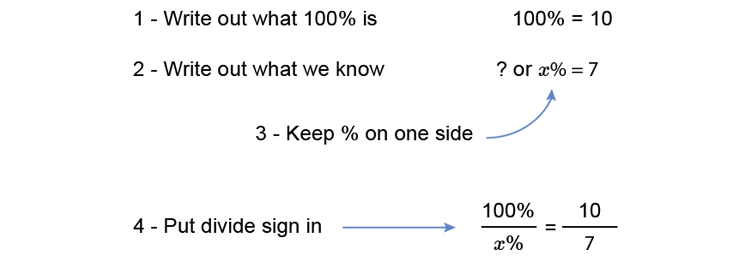# Fractions to percentages

1. Change 7/10 to a percentage.Multiply both sides by x (what you do to one side you do to the other) to get x on its own.

(100timescancelx)/cancelx=(10timesx)/7

100=(10x)/7

Multiply both sides by 7 to get x on its own.

100times7=(10xtimescancel7)/cancel7

700=10x

Divide both sides by 10 to get x on its own.

700/10=(cancel10x)/cancel10

x=700/10

x=70

Answer: x=70%